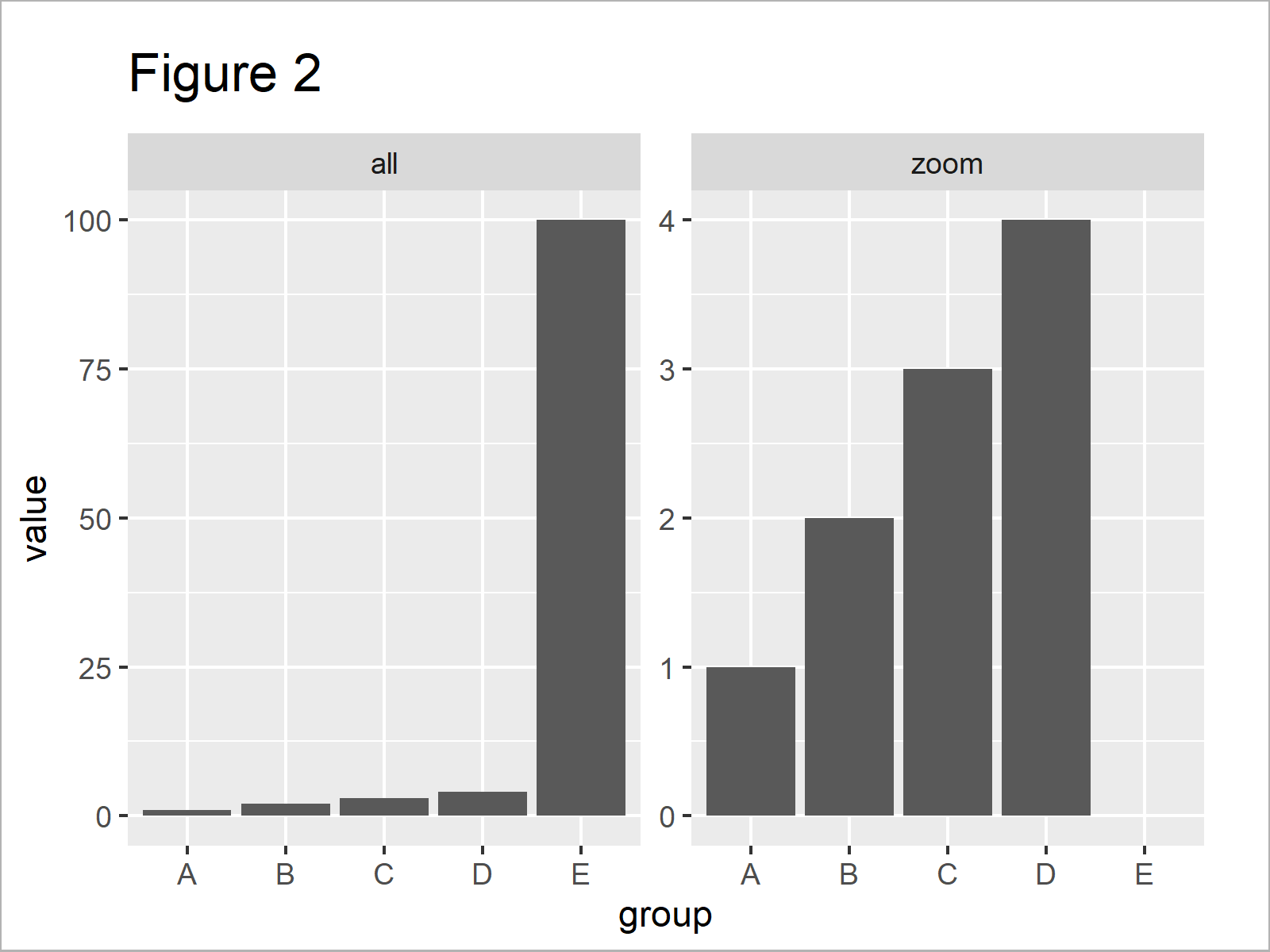# ggplot2 Barplot with Axis Break & Zoom in R (2 Examples)

In this R tutorial you’ll learn how to create a ggplot2 barchart with axis break and zoom.

The tutorial will contain the following contents:

Let’s get started:

## Example Data, Packages & Default Plot

Have a look at the following example data:

```data <- data.frame(group = LETTERS[1:5],      # Create example data
value = c(1:4, 100))
data                                          # Print example data```Have a look at the table that got returned after executing the previous R syntax. It shows that our example data is constituted of five rows and two columns, i.e. a grouping variable and the corresponding values.

To be able to draw our data with the ggplot2 package, we also have to install and load ggplot2:

```install.packages("ggplot2")                   # Install ggplot2 package

As next step, we can draw our data in a barplot:

```ggplot(data, aes(group, value)) +             # ggplot2 plot without zoom
geom_bar(stat = "identity")```As revealed in Figure 1, we have plotted a ggplot2 barchart with default axis specifications with the previous R code.

As you can see, one of the bars is much larger/higher than the other bars. For that reason, it is difficult to compare the lower bars properly.

In the following examples, I’ll show two solutions how we can handle this problem!

## Example 1: Manipulate Data to Show ggplot2 Barchart with Zoom

The following R programming syntax explains how to modify our input data frame to create a ggplot2 barplot with breaks and zoom.

Let’s modify our data:

```data_zoom <- data[data\$value <= 10, ]         # Manipulate data frame
data_zoom\$zoom <- "zoom"
data_extended <- data
data_extended\$zoom <- "all"
data_extended <- rbind(data_extended, data_zoom)
data_extended                                 # Print updated data```As shown in Table 2, the previous R code has created a new data frame that contains the same rows as our original data plus a duplicate of all rows where the values are below 10.

In addition to that, we have appended a new variable to our data frame that identifies our original data (i.e. “all”) and the appended subset (i.e. “zoom”).

We can now use this updated data frame to create a ggplot2 facet plot:

```ggplot(data_extended, aes(group, value)) +    # ggplot2 facet_wrap plot
geom_bar(stat = "identity") +
facet_wrap(~ zoom, scales = "free_y")```As shown in Figure 2, the previous R programming code has plotted a facet plot where the left plot panel shows our original data and the right panel shows only the subset of smaller values.

Note that the large bar was completely removed from the zoomed version of our plot.

The previous code worked fine. However, in the following Example I’ll show an even easier solution to our problem. So keep on reading!

## Example 2: Use ggforce Package to Show ggplot2 Barchart with Zoom

The following R programming code illustrates how to use the ggforce package to create a zoomed version of our data.

In order to use the functions of the ggforce package, we first need to install and load ggforce.

```install.packages("ggforce")                   # Install & load ggforce package
library("ggforce")```

Next, we can draw our data using the facet_zoom function provided by the ggforce package:

```ggplot(data, aes(group, value)) +             # ggplot2 facet_zoom plot
geom_bar(stat = "identity") +
geom_col() +
facet_zoom(ylim = c(0, 10))```In Figure 3 it is shown that we have created a facet zoom bargraph showing our data in a zoomed version on the left side and the original unzoomed version on the right side.

Note that this time all bars are shown in the zoomed version and the high bar was cut off according to our specification of the axis limits.

## Video & Further Resources

Would you like to learn more about ggplot2 graphics? Then you may want to watch the following video of my YouTube channel. In the video, I’m explaining the R codes of this article in the R programming language.

Please accept YouTube cookies to play this video. By accepting you will be accessing content from YouTube, a service provided by an external third party.If you accept this notice, your choice will be saved and the page will refresh.

Additionally, you might have a look at the related articles on www.statisticsglobe.com. I have published several related tutorials on topics such as ggplot2, graphics in R, and labels.

Summary: In this R programming tutorial you have learned how to draw a ggplot2 bargraph with break and zoom in the axis. Don’t hesitate to let me know in the comments, if you have any further comments or questions. Besides that, please subscribe to my email newsletter to get regular updates on new tutorials.

Subscribe to the Statistics Globe Newsletter